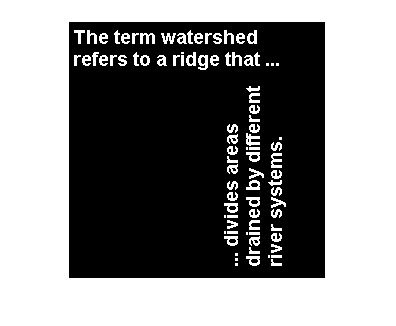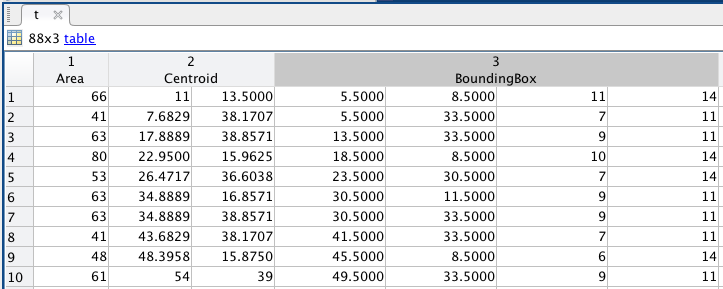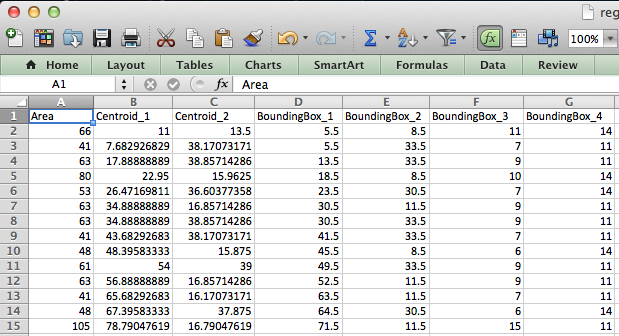# regionprops, tables, and struct2table

Today I was talking to Spandan (a regular guest blogger here) about the history of the Image Processing Toolbox function regionprops. This function is a widely-used work-horse of the toolbox. Many of my blog posts here have shown it in practice.

The function regionprops computes properties of regions of pixels. Most often, these regions correspond to individual "objects" (connected components of foreground pixels) of a binary image. Here's an example.

bw = imread('text.png');
imshow(bw)s = regionprops(bw)

s =

88x1 struct array with fields:

Area
Centroid
BoundingBox



The output is an 88x1 struct array, elements of which contain measurements for objects in the binary image. (We didn't tell regionprops what measurements to compute, so it computed Area, Centroid, and BoundingBox by default.) Here are the measurements for the 10th object.

s(10)

ans =

Area: 61
Centroid: [54 39]
BoundingBox: [49.5000 33.5000 9 11]



This form of output is convenient in some ways but inconvenient or awkward in others. For example, it requires the obscure "comma-separated-list" syntax to get all the area measurements into a single vector to call a function such as mean, min, or max.

a = [s.Area];
mean(a)

ans =

54.2386


min(a)

ans =

6


max(a)

ans =

106



In the comma-separated list syntax for struct arrays, the expression [s.Area] automatically expands into the expression [s(1).Area, s(2).Area, ..., s(end).Area]. But relatively few people recognize or understand this syntax.

Another inconvenient aspect of the struct array aspect is that it's hard to get a tabular view of all the data. As shown above, you can see the data for the 10th object by displaying s(10), but it's hard to see all the data for multiple objects. For example:

s(1:5)

ans =

5x1 struct array with fields:

Area
Centroid
BoundingBox



If I were designing regionprops from scratch today, based on the most recent version of MATLAB, I would have regionprops return a table. Tables were introduced as a new fundamental MATLAB type in R2013b. Here's a simple example to illustrate.

age = [38 43 38 40 49]';
height = [71 69 64 67 64]';
weight = [176 163 131 133 119]';
blood_pressure = [124 93; 109 77; 125 83; 117 75; 122 80];
t = table(age,height,weight,blood_pressure)

t =

age    height    weight    blood_pressure
___    ______    ______    _______________

38     71        176       124          93
43     69        163       109          77
38     64        131       125          83
40     67        133       117          75
49     64        119       122          80



t.height is an ordinary 5-by-1 vector of heights.

t.height

ans =

71
69
64
67
64



t.blood_pressure is an ordinary 5-by-2 matrix. (Table "variables" are often column vectors, but they can have any size as long as the number of rows is the same as the height of the table.)

t.blood_pressure

ans =

124    93
109    77
125    83
117    75
122    80



We are excited about the introduction of tables in MATLAB, and we think they will gradually be used in more and more situations where previously people used struct arrays.

Such as regionprops.

So what to do?

Well, the function struct2table comes in very handy here! As you might have guessed from the name, it automatically converts a struct array into a table.

Try it:

t = struct2table(s)

t =

Area         Centroid         BoundingBox
____    __________________    ____________

66         11        13.5    [1x4 double]
41     7.6829      38.171    [1x4 double]
63     17.889      38.857    [1x4 double]
80      22.95      15.963    [1x4 double]
53     26.472      36.604    [1x4 double]
63     34.889      16.857    [1x4 double]
63     34.889      38.857    [1x4 double]
41     43.683      38.171    [1x4 double]
48     48.396      15.875    [1x4 double]
61         54          39    [1x4 double]
63     56.889      16.857    [1x4 double]
41     65.683      16.171    [1x4 double]
48     67.396      37.875    [1x4 double]
105      78.79       16.79    [1x4 double]
66       76.5          39    [1x4 double]
68     93.529      39.265    [1x4 double]
92        100      16.696    [1x4 double]
41     106.68      38.171    [1x4 double]
68     113.53      17.265    [1x4 double]
6        114        31.5    [1x4 double]
33        114          39    [1x4 double]
85     123.24      38.259    [1x4 double]
48      121.4      15.875    [1x4 double]
63     129.89      16.857    [1x4 double]
100     134.87       40.86    [1x4 double]
41     138.68      16.171    [1x4 double]
63     145.89      38.857    [1x4 double]
61        149          17    [1x4 double]
80     159.95      15.963    [1x4 double]
85     163.26      185.76    [1x4 double]
6      156.5         195    [1x4 double]
6      156.5         211    [1x4 double]
85     163.26      218.76    [1x4 double]
48      159.4      37.875    [1x4 double]
61        164         107    [1x4 double]
68     164.26      117.47    [1x4 double]
63     163.86      129.11    [1x4 double]
41     163.17      139.32    [1x4 double]
68     164.26      147.47    [1x4 double]
61        164         164    [1x4 double]
63     163.86      175.11    [1x4 double]
33        164         195    [1x4 double]
54     163.44         203    [1x4 double]
33        164         211    [1x4 double]
80     167.95      37.962    [1x4 double]
9        168         233    [1x4 double]
9        168         238    [1x4 double]
9        168         243    [1x4 double]
63     171.89      16.857    [1x4 double]
68     180.53      39.265    [1x4 double]
48     184.88      67.604    [1x4 double]
106      183.6      118.53    [1x4 double]
6      178.5         127    [1x4 double]
85     185.26      134.76    [1x4 double]
85     185.26      164.24    [1x4 double]
85     185.26      179.76    [1x4 double]
6      178.5         212    [1x4 double]
85     185.26      238.76    [1x4 double]
85     184.24      16.259    [1x4 double]
72     185.68      76.653    [1x4 double]
63     185.86      88.111    [1x4 double]
41     185.17      98.317    [1x4 double]
63     185.86      107.11    [1x4 double]
33        186         127    [1x4 double]
74      187.2      152.31    [1x4 double]
63     185.86      192.11    [1x4 double]
72     185.68      203.65    [1x4 double]
33        186         212    [1x4 double]
68     186.26      219.47    [1x4 double]
41     185.17      230.32    [1x4 double]
48      188.4      37.875    [1x4 double]
9        199          43    [1x4 double]
48     206.88       161.6    [1x4 double]
6      200.5         235    [1x4 double]
9        204          43    [1x4 double]
61        208         125    [1x4 double]
105     207.79      139.21    [1x4 double]
63     207.86      153.11    [1x4 double]
61        208         170    [1x4 double]
74      209.2      181.31    [1x4 double]
61        208         192    [1x4 double]
41     207.17      207.32    [1x4 double]
63     207.86      216.11    [1x4 double]
54     207.44         227    [1x4 double]
33        208         235    [1x4 double]
41     207.17      242.32    [1x4 double]
9        209          43    [1x4 double]
9        212         117    [1x4 double]



Immediately, you can see that the data is much more easily visible with a table. You get scrolling and editing if you open t in the Variable Editor.You can plot directly with the data in a table variable:

imshow(bw)
hold on
plot(t.Centroid(:,1),t.Centroid(:,2),'r*')
axis([.5 100.5 .5 100.5])
hold offLogical indexing comes in handy for filtering objects based on various criteria. For example, the following code removes objects with area less than 10 from the table.

t(t.Area < 10,:) = [];


And you can easily export tables to various formats. Here is our table exported to a .CSV file and then read into Excel.

writetable(t,'regionprops.csv');I encourage everyone who has used regionprops to play around with tables (assuming you have MATLAB R2013b) to see how they might be useful in your own work.

Published with MATLAB® R2013b

|